# Cryptocurrency scalping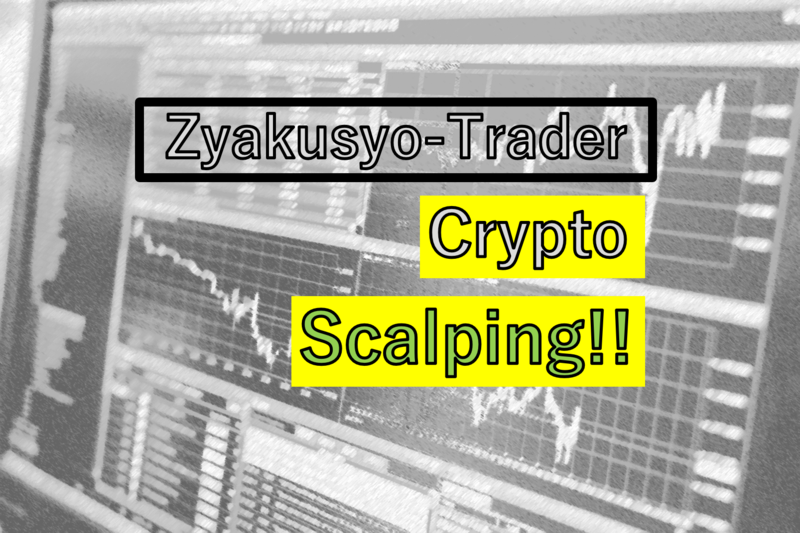Should cryptocurrency scalping is recommended?? Let's study!!

Thanks for coming!!

Have a precious time here(^_^)/

I can not perform a leveraged trade because I do not have enough money to do so.*1

Here, I would like to study whether we can scalp from spot BTC.

### BitCoin(BTC)

#### Can we scalp from spot BTC??

The money I can use is just 20,000JPY.

Then, can I scalp??

##### Case#1．Scalp from 1-minute Chart

Suppose if I bought 0.02BTC.

Taking profit when the rate ascends 500JPY, and stop when it descends 750JPY.

In this case, the profit is about 10JPY, and the loss is approximately 15JPY.

Besides, you should consider a commission.

The bitFlyer costs 0.00003BTC for 0.02BTC.

When JPY/BTC is 1,000,000, that commission is roughly 30JPY.

To summarize, you shouldn't scalp from 1-minute Chart.

If you want to, the ATR should be more than 1500JPY.

How long is the ATR above 1500JPY?

Below is an example of ATR.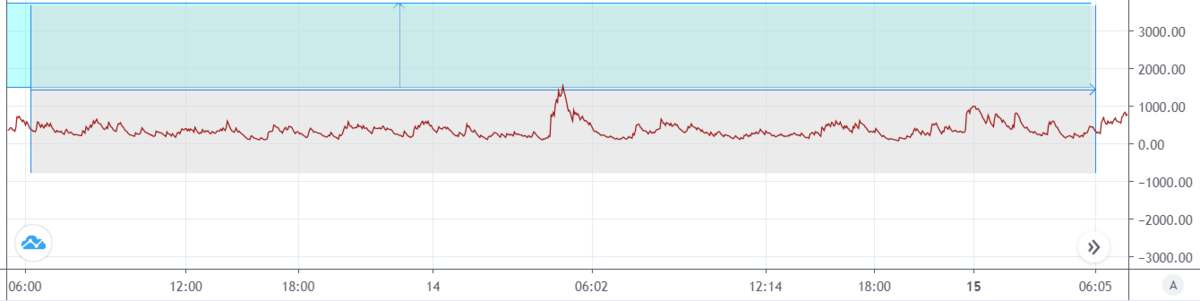The sky-blue zone is where the ATR is above 1500JPY.

It rarely reaches. I should give up scalping from 1-minute Chart.

##### Case#2．Scalp from 5 minutes Chart

Same as #1, we need the ATR above 1500JPY.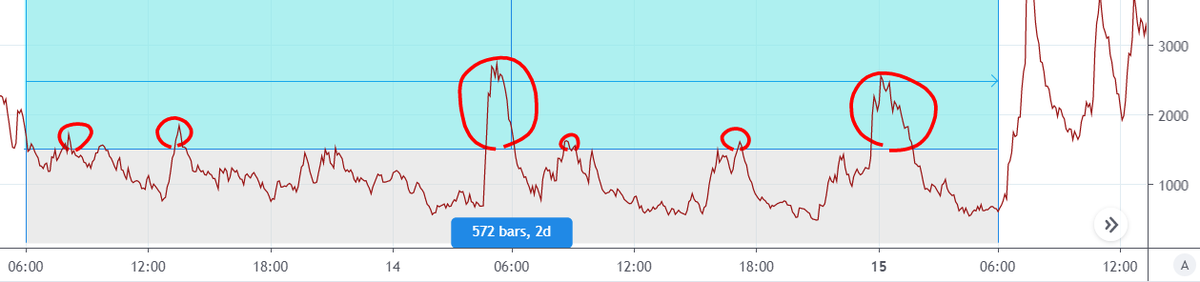It reached more than the former one, but it needs more reach.

##### Case#3．Scalp from 15 minutes Chart*3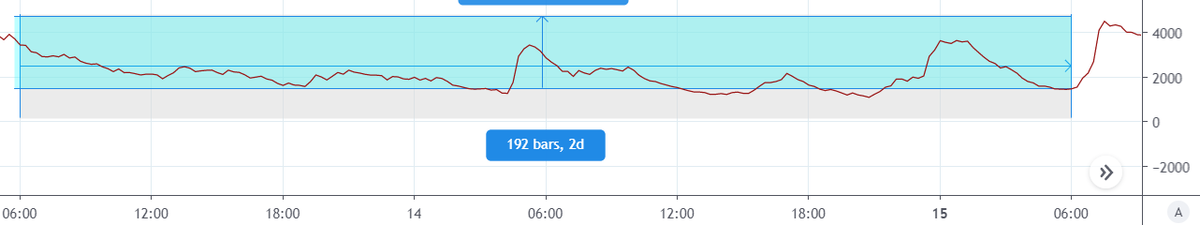Seems good!!

In this case, the average ATR is about 2500JPY, isn't it?

For example, suppose taking profit at +2500JPY and cutting the loss at -4000JPY.

The profit will be 50JPY, and the loss will be 80JPY for the cost of 30JPY.

To put in a simple way, this is a lottery that costs 30JPY.

If we win, get 50JPY, and if we lose, miss 80JPY.

#### Conclusion

If you want to scalp from BTC, the optimal chart is 10 minutes.

However, the high amount of commission deters us from doing scalping.

### Can we scalp from Etherium?

Think in the same way as BTC.

If we buy 0.8ETH, the commission is 0.0012ETH.

BitFlyer costs me 30JPY when JPY/ETH is 25,000.

That's why I want the ATR as much as 40JPY.

#### Case#1．1-minute Chart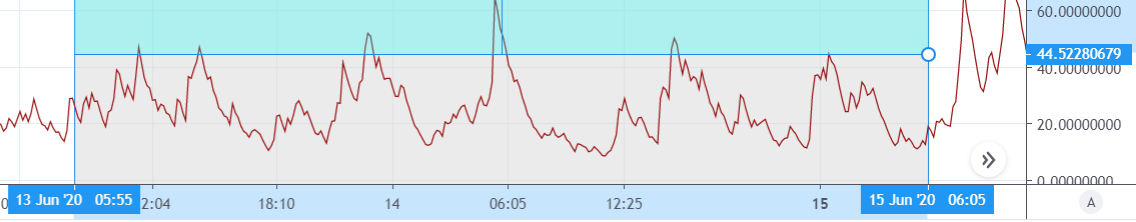It's out of question. NEXT!

#### Case#2．5 minutes Chart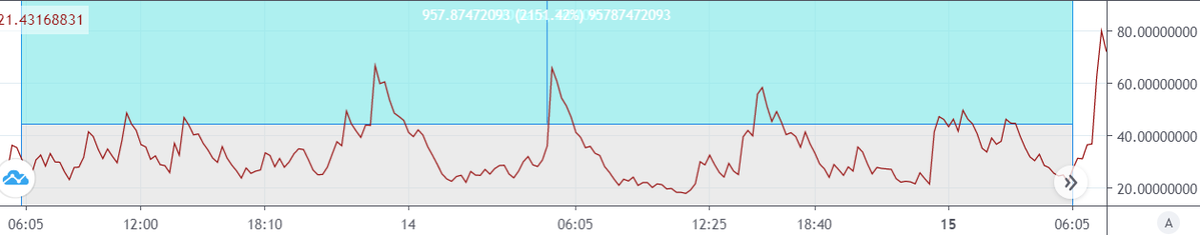It seems OK, but far from perfect.

#### Case#3．15 minutes Chart*4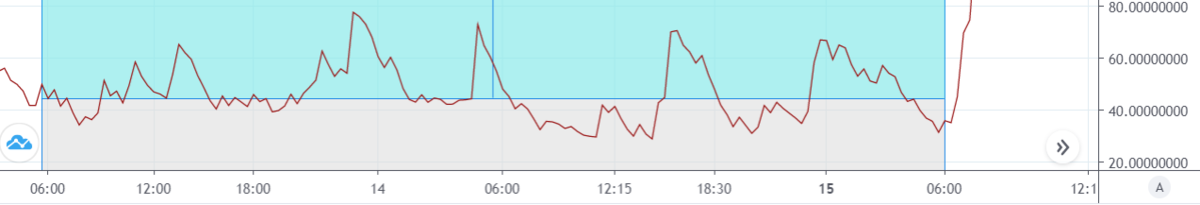That's good!! I can expect the ATR roughly 60.

I take profit at +60JPY and cut a loss at -90JPY with a commission of 30JPY.

### What crypto should we chose for scalping?

In bitFlyer, chosing from BTC or ETH, I recommend ETH.

Not only bitFlyer but also other brokers, the commission of crypto takes much.

### Where is the break-even？？

#### If you like math, see below.

Let R be an original rate, and a be the amount of rate ascended from R.

Let q be the amount you bought, then 0.0015q is a commission.

Let the amount you buy and sell is equal, then the amount you sell is

aq-(R+(R+a))*0.0015q

=(0.9985a - 0.0030R) q

q>0, so the break-even point meets

0.9985a=0.0030R

R = 332.833a

R=333a

#### To conclude

There will be a loss when the original rate exceeds 333 times of the rate changed.

##### If you buy at the rate of 1,000,000･･･

The break even point is about 3000. (1,000,000/333)

#### What if the commission changes??

Let c the new commission, the new break-even point will be

0.9985a = 2cR

so R =(0.49925/c) a

*1:Even if I have a lot of money, I don't do this. Cryptocurrency has a substancial amount of risk.

*2:The maximum ATR is 2000JPY.

*3:It's similar to 10 minutes Chart.

*4:It's also similar to 10 minutes Chart

html text val